Function Repository Resource:

# UnitSystemTransform

Transform a quantity into a different unit system

Contributed by: Peter Cullen Burbery
 ResourceFunction["UnitSystemTransform"][quantity,unitsystem] transforms quantity into a product of combinations of the basis quantities of unitsystem.

## Details

The following unitsystems are currently supported:
 "PlanckUnits" the Planck system without electrical units "NaturalUnits" an extension to the Planck system that spans electrical units with the Von Klitzing constant "SIDefiningConstants" the seven defining constants of the SI {q1,q2,…} list of Quantity expressions with appropriate units "StoneyUnits" the Stoney unit system, similar to the Planck unit system
The output of ResourceFunction["UnitSystemTransform"] is a list, as sometimes the basis will have multiple equivalent dimensional combinations. For example, if the basis quantities span the dimensions but aren’t linearly independent, there will be at least two possible combinations.

## Examples

### Basic Examples (6)

Convert to the Planck unit system:

 In:=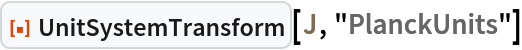Out=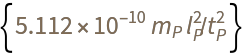In:=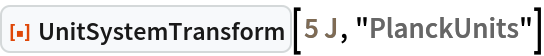Out=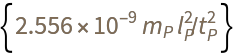Convert to a set of natural units:

 In:=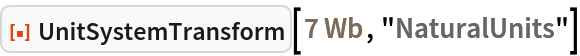Out=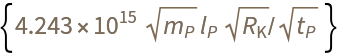Convert to the SI-defining constants:

 In:=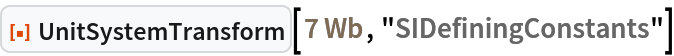Out=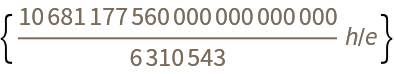Convert to Stoney units:

 In:=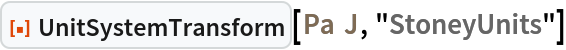Out=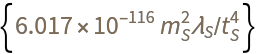Convert to a custom combination of quantities:

 In:=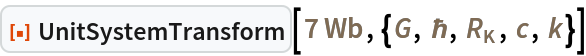Out=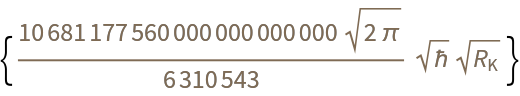Find multiple equivalent forms:

 In:=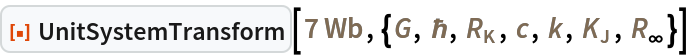Out=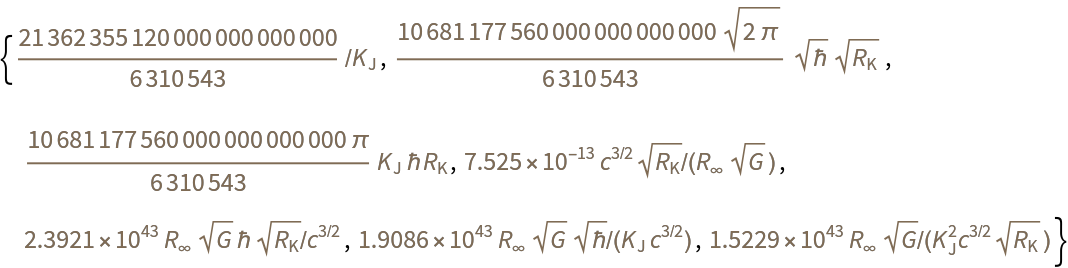### Possible Issues (1)

If the basis doesn't span the dimensions of the input, the output will be an empty list:

 In:=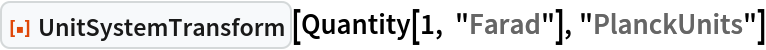Out=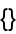Peter Burbery

## Version History

• 1.0.0 – 07 June 2023